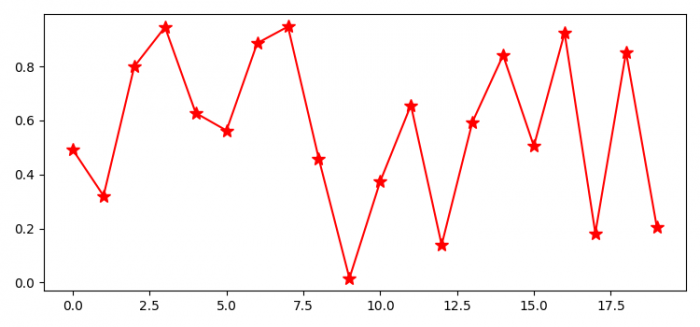# How to import Matplotlib in Python?

First of all, make sure you have python and pip preinstalled on your system. To check Python version, type

python --version

To check pip version, type

pip −V

Then, run the following pip command in the command prompt to install Matplotlib.

pip install matplotlib

To verify that matplotlib is successfully installed on your system, execute the following command in the command prompt.

import matplotlib
matplotlib.__version__

If matplotlib is successfully installed, the version of matplotlib will be displayed.

Now, let us import Matplotlib and plot some random data points.

## Steps

• Import matplotlib.
• Set the figure size and adjust the padding between and around the subplots.
• Create random data points, x.
• Plot x using plot() method.
• To display the figure, use show() method.

## Example

from matplotlib import pyplot as plt
import numpy as np

plt.rcParams["figure.figsize"] = [7.50, 3.50]
plt.rcParams["figure.autolayout"] = True

x = np.random.rand(20)
plt.plot(x, '*-', color='red', markersize=10)

plt.show()

## Output